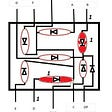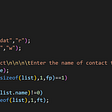# Understanding Linked List Cycles via Floyd’s Algorithm

This week I had my horizons stretched to new lengths while being mock-interviewed. I was asked to solve an algorithm which accepts an unsorted array of numbers increasing by one that contains one duplicate and returns the duplicate number. At first I was asked to do it with the highest time complexity, which I promptly solved using a nested for-loop. Anticipating the next question, I began on the solution which is completed involves using a hash map (or object) to collect the number of times an integer appears within the array and returns the key of the instance with a value of two. After completing this I was asked to solve the problem in O(n) time and O(1) space, to which I thought and thought about but ultimately had no idea about. We moved on but I was still perplexed over the possibility of this solution, so in my free time, dove head first into what is known as Floyd’s Algorithm.

# WHAT IS A LINKED LIST?

Before getting into this, I think it’s important to understand what a linked list is. A linked list is a sequence of data structures (nodes) which are joined together by a series of pointers. The first position points to a “head”, and the final points to a “null” value. This is fairly similar to an array, except the use of pointers contains more possibilities as the way the pointers interact with the data can create a number of scenarios whereas arrays are only referenced by index.

# WHAT IS A CYCLE?

Referring to the above diagram, a cycle in a linked list is when the would-be final value points to an earlier node in the list. This creates a closed system where the earlier position moves forward to the end over and over again.

# WHAT IS FLOYD’S ALGORITHM?

Floyd’s algorithm essentially creates two iterators which run through the linked list at different speeds, generally one twice as fast as the other. This is why it is sometimes referred to as the “Tortoise & the Hare” algorithm. If a cycle exists, they will eventually meet on the same node and thus return either a boolean value if the algorithm is asking whether a cycle exists, the point at which they meet, or in the case which I was asked, the point where the cycle begins, which has a lot going into it.

# HOW CAN IT DETECT THE STARTING POINT?

The way this clicked for me was understanding that the indexes of the nodes can be used to point to one another. This graphic helped me understand it possibly more than anything:

The values are in orange and the indexes are in purple. You will see the head position begins at the index of zero. The value of index zero “points” to the next index, or becomes the next value, which is index four. The value of index four points to the next index, which is six, and so on. The value of two exists at both index six and nine, but six makes the first appearance and so that becomes the entry point of the cycle. This pattern repeats until two appears again at index nine, which points back to index two and around and around we go. By using two constituent iterators moving at separate speeds as in Floyd’s algorithm, we can guarantee they will meet at some point, as the Moon eclipses the Sun from our perspective, but how to determine where the entry point begins requires some math.

So, imagining that two iterators moving at two different speeds (one twice as fast as the other) have run and met around the cycle, we can extract the starting point like so. With the shape of the linked list being a loop with a head, we can call the stretch before the cycle “x”, and then set the distance before the meeting point as “y” and the distance after the meeting point as “z”. The length of the cycle will appear as such:

`l = y + z`

If we let “f” equal the distance traveled by the faster iterator and “s” the distance traveled by the slower iterator, it will be:

`f = x + c₁l + ys = x + c₂l + y`

Since “f” is being used as twice the speed of “s”, we can represent it like this:

`2x + 2c₂l + 2y = x + c₁l + y`

With the “x”s and “y”s on the same side it looks like this:

`2x + 2y - x - y = c₁l - 2c₂l`

Or:

`x + y = (c₁ - 2c₂)l`

To get the distance before the meeting point, we have to get “x” (the point before the cycle) on one side. If we subtract “y” from the left side we get:

`x = (c₁ - 2c₂)l - y`

This means the distance before the cycle is equal to a certain number of loops around the cycle minus the distance of the meeting point. This is the same as:

`x = (c₁ - 2c₂ - 1)l + 1 - y`

Which is the same as:

`x = c₃l + l - y`

Remember l = y + z so we can write it like this:

`x = c₃l y + z - y`

Which can be reduce to…

`x = c₃l + z`

Just like that *whew*! We have the formula for the length of x. Now that we’ve looked at what is taking place underneath the hood, let’s translate this to code.

# SOLUTION

The most common solution for this algorithm is available to find all over the web, but I will include the python solution here just for reference:

`class Solution(object):  def findDuplicate(self, numbers):  tortoise = numbers  hare = numbers  while True:    tortoise = numbers[tortoise]    hare = numbers[numbers[hare]]    if tortoise == hare;      break  pointer1 = numbers  pointer2 = tortoise  while pointer1 != pointer2:    pointer1 = numbers[pointer1]    pointer2 = numbers[pointer2]  return pointer1`

I hope this helps understand this brilliant programming concept!

Until next time…

--

--

--

## More from Lucas Thinnes

Programmer / Artist / Believer

Love podcasts or audiobooks? Learn on the go with our new app.

## How to automate deployment in a React App to Github Pages using Github Actions## Recapitulation of GameYoo PROJECT AMA event held at AMA LOVERS CLUB.## Top Software development companies in the UK — Reviews## Hornet 0.4.0 Release Summary## What is satisfaction for a programmer?## JavaScript Algorithms## Okafor Ebubechukwu chukwuamaka## Lucas Thinnes

Programmer / Artist / Believer

## More from Medium